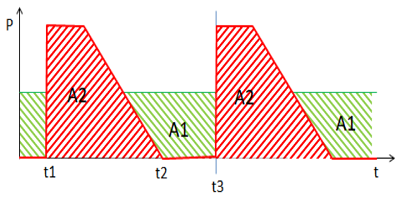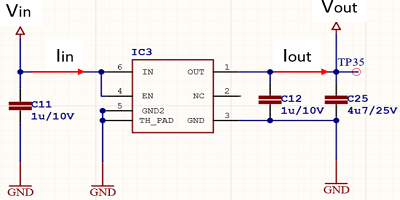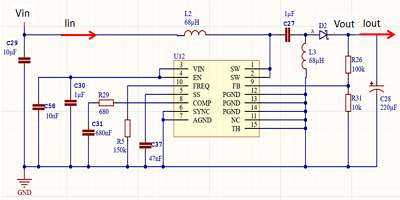﻿ 电路设计功耗问题--上海韬放电子科技有限公司

### 电路设计功耗问题ƞ= Pout /引脚Pᴬᴱ=Iᴿᴹˢ*UᴿᴹˢPloss = Pin – Pout

= Vin *输入-Vout * Iout

= 5V * 0.14A-3.6V * 0.14A

= 0.7W – 0.504W

= 0.196

ƞ= 0.504W / 0.7W = 0.72Pout = Vout * Iout

Vout * Iout =ƞ*引脚

13.5V * 0.08A =ƞ* 20V * Iin

1.08W =ƞ* 20V * Iin

Iin = 1.08W /0.85 * 20V= 64mA

Ploss =引脚-Pout = 0.064A * 20V-0.08A * 13.5V = 1.28W-1.08W = 0.2W

Iin = 13.5V * 0.08A / 0.85 * 10V = 0.129A

Ploss =引脚-Pout = 0.129A * 10V-0.08A * 13.5V = 1.29W-1.08W = 0.21W